• Mass_Univariate_ERP_Toolbox MATLAB函数用于分析和可视化对与事件相关的潜在数据进行的大量t检验。 Mass Univariate ERP工具箱是可免费使用的一组MATLAB函数，用于执行事件相关电位（ERP）的质量单变量分析，事件...
• 本书介绍多种不同的离散分布。从简单的Bernoulli, Binomial 到 Hypergeometric, Negative Binomial, Poisson 到之后更加复杂的分布都有详细的介绍。是一本十分详细的参考书。
• SPSS Data Analysis for Univariate, Bivariate, and Multivariate Statistics By 作者: Daniel J. Denis ISBN-10 书号: 1119465818 ISBN-13 书号: 9781119465812 Edition 版本: 1 出版日期: 2018-09-25 pages 页数:...Analytics
• UCR/UEA是时间序列界的“Imagnet”，单变量TSC存档于2018年重新发布，包含128个数据集，Weka格式的ARFF文件（和.txt文件）（约500 MB）第二部分
• 这个 matlab 函数有助于使用 Pettitt 检验在单变量时间序列数据集中找到非参数单一变化点。matlab
• 时间序列界的“Imagnet”，发文章必跑数据集。大约有128个数据集。此数据集为单变量TSC存档于2018年重新发布，包含128个数据集，sktime格式的ts文件。
• Continuous Univariate Distributions, Vol. 2Continuous
• Continuous Univariate Distributions, Vol.Continuous
• ## SAS univariate过程

千次阅读 2018-07-03 16:32:52
EDA(探索性数据分析)最常用的过程步之一就是PROC UNIVARIATE。 首先先看一个最简单的PROC UNIVARIATE程序： PROC UNIVARIATE DATA=SASHELP.FISH; WHERE SPECIES='Bream'; VAR HEIGHT; RUN; 上述代码得到的结果...

EDA(探索性数据分析)最常用的过程步之一就是PROC UNIVARIATE。
首先先看一个最简单的PROC UNIVARIATE程序：

PROC UNIVARIATE DATA=SASHELP.FISH;
WHERE SPECIES='Bream';
VAR HEIGHT;
RUN;

上述代码得到的结果有：矩、位置和可变形的基本测度、位置检验、分位数、极值观测。具体如下：解读：首先看第一张表——矩，其中N=35代表该进入分析变量有35条观测，偏度约0.2稍微有点负偏态（右偏），变异系数12（不大也不小，无法得出结论），峰度系数-0.6（低峰后尾）；
第二张表：位置和变异性的基本测度，其中位置的统计量有均值（15.1），中位数（14.9）还是比较接近，所以近似对称分布。变异性即数据的差异性程度（标准差越大差异越大。）

PROC UNIVARIATE DATA=SASHELP.FISH;
WHERE SPECIES='Bream';
VAR HEIGHT;
HISTOGRAM ;
RUN;

若加了一个HISTOGRAM则增加了一个直方图，如下：如加一个NORMAL选项则在直方图上面加了一根拟合后的正态分布图，并且还增加了拟合正太分布的参数估计、拟合优度、分位数，这里的括号中的意思可理解为均值（MU）和标准差（SIGMA）的值为原始数据本身的均值和标准差；

PROC UNIVARIATE DATA=SASHELP.FISH;
WHERE SPECIES='Bream';
VAR HEIGHT;
HISTOGRAM /NORMAL(MU=EST SIGMA=EST);
RUN;PROC UNIVARIATE DATA=SASHELP.FISH;
WHERE SPECIES='Bream';
VAR HEIGHT;
HISTOGRAM /NORMAL(MU=EST SIGMA=EST) KERNEL;
RUN;

加了一个KERNEL则在直方图中加了一根实际数据核分布的密度曲线，如下图：PROC UNIVARIATE DATA=SASHELP.FISH PLOT;
WHERE SPECIES='Bream';
VAR HEIGHT;
HISTOGRAM /NORMAL(MU=EST SIGMA=EST) KERNEL;
RUN;

上述加了一个plot选项，在结果中增加了分析变量数据的分布图、盒形图、以及概率图，如下：由概率图看出，散点分布的直线上下，还算是比较重合，所以该数据近似服从正态分布的。

暂时就会这么多了。。以后再补。

展开全文• 本文想给大家分享的是《A Univariate Bound of Area Under ROC》论文。 还是那句话水平有限，大家多多包涵，非常欢迎任何形式的讨论，大家共同学习共同进步。码字不易，喜欢就请大家点赞、收藏、转发三连吧！ 蟹蟹...
本文想给大家分享的是《A Univariate Bound of Area Under ROC》论文。

还是那句话水平有限，大家多多包涵，非常欢迎任何形式的讨论，大家共同学习共同进步。码字不易，喜欢就请大家点赞、收藏、转发三连吧！蟹蟹大家的支持，后面我也会把整个系列总结到一起更新到公众号！欢迎大家关注，转发，分享三连！ 需要下载原论文的，可以关注公众号，后台回复“UBAUC”，下载论文！

Abstract & Intro
ROC下面积（AUC）是二元分类和二部排序问题的一个重要指标。然而，很难将AUC作为直接优化的学习目标(0-1损失是离散的)，所以现有的大多数算法都是基于对AUC的替代损失进行优化。替代损失的一个重大缺点就是他们需要对训练数据的成对比较，这导致运行时间慢，并且增加了用于在线学习的本地存储。 本文提出了一个基于AUC risk的新的替代损失，它并不需要成对比较，但仍然可以对预测进行排序。作者进一步证明了，排序操作可以被避免，并且基于该代理项得到的学习目标在时间和存储上都具有线性复杂度。 最后，实验验证了基于代理损失的AUC优化在线算法和批处理算法的有效性。
Problem Definition

展开全文机器学习 人工智能 数据挖掘 深度学习
• matlab代码中向量的点乘线性回归 预测连续值（实值）... 代码在Octave版本4.2.1上成功执行 线性回归 在这个项目中，我实现了线性回归，并看到它在...ex1data1.txt-具有一个变量的线性回归数据集（Univariate_Linear_Re
• Compare univariate, multivariate, and multiview embeddings Forecasting skill improves if we use multivariate or multiview embedding compared with univariate embedding (Fig. 6d). rhos (simp_C1[]$... Load package and time series Time series are generated according to a 5-species model (Resource, Consumer1, Consumer2, Predator1, Predator2) following Deyle et al. (2016). Note that the model is started with a burn-in period to ensure the dynamics relax to attractor manifold (see Deyle et al. 2016). ## Load package and data library(rEDM) d <- read.csv("ESM5_Data_5spModel.csv")  Univariate embedding (Sugihara & May 1990) In this demonstration, we want to forecast dynamics of Consumer1 (C1). Information (history) encoded in the dynamics of C1 is used to forecast the dynamics of C1. # Please reduce the number of data points if the calculation needs long time data_used <- 1:1000 # Specify the length of time series to be used to reconstruct state space (Library length) lib_point <- c(1,floor(max(data_used)/2)) # Specify which points will be predicted based on the reconstructed state space pred_point <- c(floor(max(data_used)/2)+1, max(data_used)) # Time series of C1 is normalized C1 <- as.numeric(scale(d[data_used,'C1'])) # Estimate the best embedding dimension simp_C1_tmp <- simplex(C1, E=1:10, silent = T) plot(simp_C1_tmp$E, simp_C1_tmp$mae, type="l", xlab="E", ylab="MAE") # Best E = 3 bestE_C1 <- simp_C1_tmp[which.min(simp_C1_tmp$mae),"E"]

# Perform univariate simplex projection
# We need to specify time series (C1), embedding dimension (E), library length (lib), predictee (pred) and which output we need (stats_only). If you do not want to see warning message, "silent" option should be set as "T".
simp_C1 <- simplex(C1, E=bestE_C1, lib=lib_point, pred=pred_point, stats_only = F, silent = T)
C1_pred_uni <- simp_C1[]$model_output$pred
C1_obs_uni <- simp_C1[]$model_output$obs
plot(C1_obs_uni, C1_pred_uni, xlab="Observed", ylab="Predicted")

上述代码是论文原版提供的，运行中会报错，参考原始资料rEDM: An R package for Empirical Dynamic Modeling and Convergent Cross Mapping，进行改正
simp_C1 <- simplex(C1, E=bestE_C1, lib=lib_point, pred=pred_point, stats_only = F, silent = T)
C1_pred_uni <- simp_C1[]$model_output$pred
C1_obs_uni <- simp_C1[]$model_output$obs
plot(C1_obs_uni, C1_pred_uni, xlab="Observed", ylab="Predicted")

修改为：
simp_C1 <- simplex(C1, E=bestE_C1, lib=lib_point, pred=pred_point, stats_only = F, silent = T)
C1_pred_uni <- simp_C1$model_output[]$pred
C1_obs_uni <- simp_C1$model_output[]$obs
plot(C1_obs_uni, C1_pred_uni, xlab="Observed", ylab="Predicted")
abline(0,1) # add 1:1 line
The forecast skill (ρ) is 0.970 when we use univariate embedding (Fig. 6a in the main text).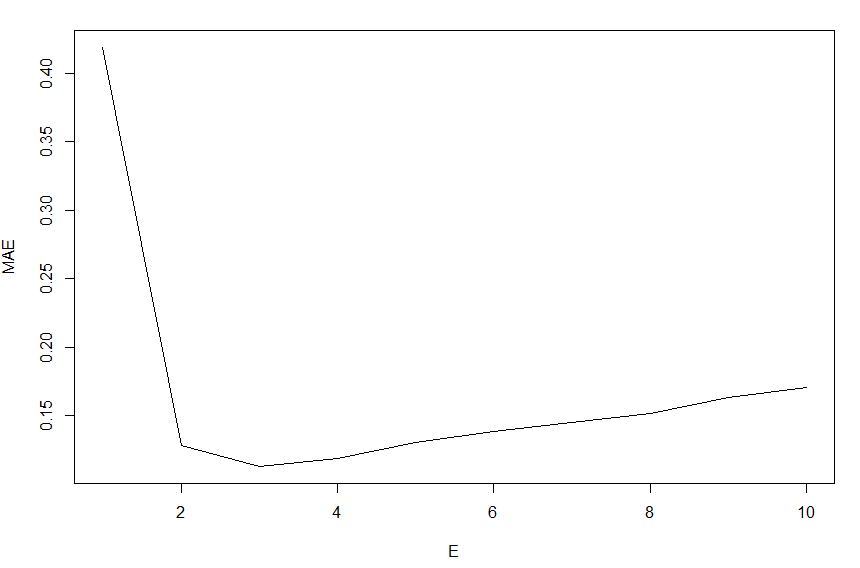最佳embedding dimension为3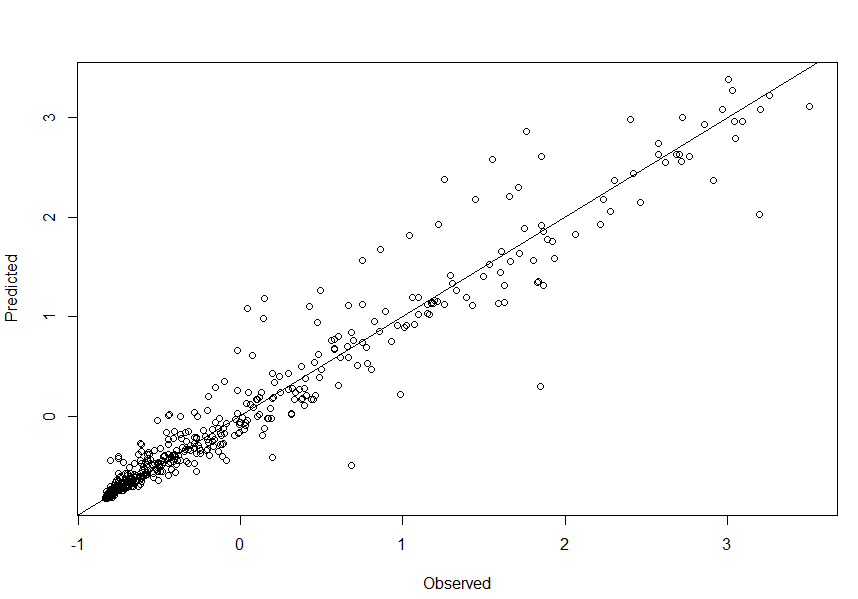Multivariate embedding (Deyle & Sugihara 2011, Deyle et al. 2013)
In this demonstration, we want to forecast the dynamics of C1. In the 5-species model, P1 and R are directly related to C1. Thus, we used C1, R and P1 for multivariate embedding.
# Make multivariate embedding
Embedding <- c("C1", "R", "P1")
block <- d[,Embedding]

# Normalize data
block <- as.data.frame(apply(block, 2, function(x) (x-mean(x))/sd(x)))

# Do multivariate simplex projection using block_lnlp() function
# We need to specify time series, method (simplex or s-map), library length (lib), predictee (pred) and which output we need (stats_only).
mult_simp_C1 <-  block_lnlp(block[data_used,], method = "simplex", lib = lib_point, pred = pred_point,
stats_only = F, silent = T)
C1_pred_mult <- mult_simp_C1$model_output[]$pred
C1_obs_mult <- mult_simp_C1$model_output[]$obs
plot(C1_obs_mult, C1_pred_mult, xlab="Observed", ylab="Predicted")

原代码同样有问题，参考上述进行修改。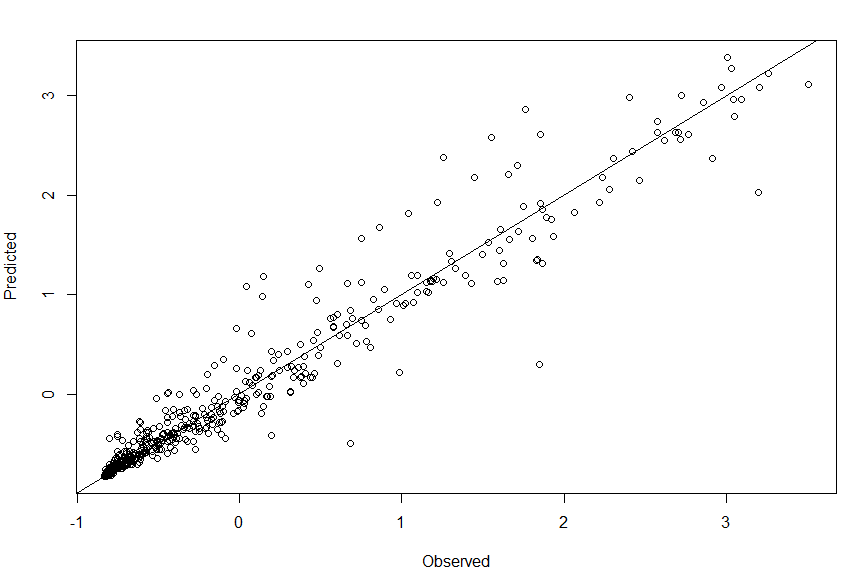The forecast skill (ρ) is 0.987 when we use maltivariate embedding (Fig. 6b).
Multi-view embedding (Ye & Sugihara 2016)
Multi-view embedding combines multiple embeddings and leverage information of many embeddings.
# Do multiview forecasting using multiview() function
# We need to specify time series, library length (lib), predictee (pred) and which output we need (stats_only).
multiview_C1 <- multiview(block[data_used,], lib = lib_point, pred = pred_point, stats_only = F, silent = T)
C1_pred_multv <- multiview_C1$model_output[]$pred
C1_obs_multv <- multiview_C1$model_output[]$obs
plot(C1_obs_multv, C1_pred_multv, xlab="Observed", ylab="Predicted")

原代码同样有问题，参考上述进行修改。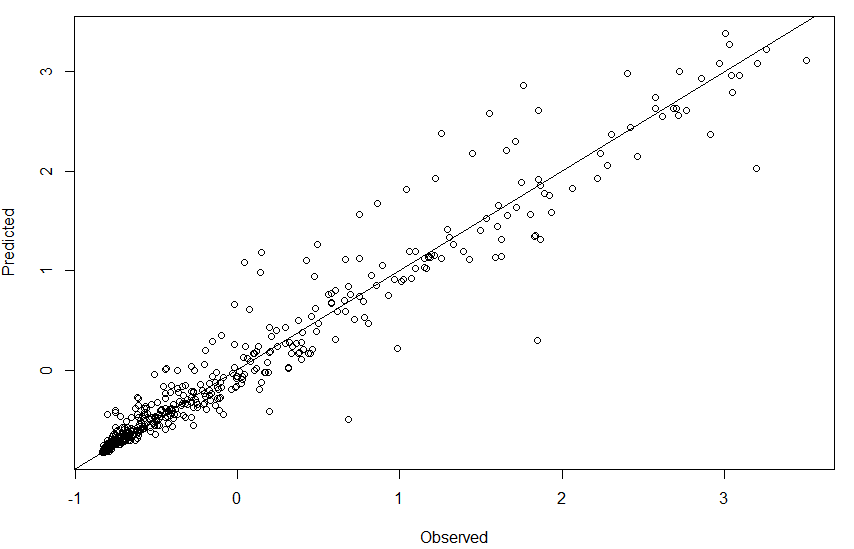The forecast skill (ρ) is 0.989 when we use multiview embedding (Fig, 6c).
References
Deyle E, Sugihara G (2011) Generalized theorems for nonlinear state space reconstruction. PLoS ONE 6: e18295.(PDF)
Deyle ER, Fogarty M, Hsieh CH, Kaufman L, MacCall AD, Munch SB, Perretti CT, Ye H, Sugihara G (2013) Predicting climate effects on Pacific sardine. Proc Natl Acad Sci USA 110: 6430-6435. DOI: 10.1073/pnas.1215506110(PDF)
Sugihara G, May R, Ye H, Hsieh CH, Deyle E, Fogarty M, Munch S (2012) Detecting causality in complex ecosystems. Science 338: 496-500. DOI: 10.1126/science.1227079(PDF)
Ye H, Sugihara G (2016) Information leverage in interconnected ecosystems: Overcoming the curse of dimensionality. Science 353: 922.(PDF)
展开全文• linear constraint for simplex methodscontraint
• sklearn官方文档:Univariate Feature Selection sklearn官方文档:Comparison of F-test and mutual information sklearn ApacheCN 官方翻译 转载于:https://www.cnblogs.com/fonttian/p/9162731.html

sklearn中的单变量特征选择
单变量的特征选择是通过基于一些单变量的统计度量方法来选择最好的特征，比如卡方检测等。Scikit-learn 将单变量特征选择的学习器作为实现了 transform方法的对象：
sklearn中实现的方法有:
SelectKBest 保留评分最高的 K 个特征SelectPercentile 保留最高得分百分比之几的特征对每个特征应用常见的单变量统计测试: 假阳性率（false positive rate） SelectFpr, 伪发现率（false discovery rate） SelectFdr , 或者族系误差（family wise error） SelectFwe 。GenericUnivariateSelect 允许使用可配置方法来进行单变量特征选择。它允许超参数搜索评估器来选择最好的单变量特征。
这些对象将得分函数作为输入，返回单变量的得分和 p 值 （或者仅仅是 SelectKBest 和 SelectPercentile 的分数）:
对于回归: f_regression , mutual_info_regression对于分类: chi2 , f_classif , mutual_info_classif
这些基于 F-test 的方法计算两个随机变量之间的线性相关程度。另一方面，mutual information methods（mutual information : 互信息）能够计算任何种类的统计相关性，但是作为非参数的方法，互信息需要更多的样本来进行准确的估计。
稀疏数据的特征选择
如果你使用的是稀疏的数据 (例如数据可以由稀疏矩阵来表示),  chi2 , mutual_info_regression , mutual_info_classif 可以处理数据并保持它的稀疏性。
以上文字主要来自于ApacheCN的sklearn0.19的用户手册的翻译,略有修改
例子
简单使用
from sklearn.datasets import load_iris
from sklearn.feature_selection import SelectKBest,SelectPercentile
from sklearn.feature_selection import chi2
X, y = iris.data, iris.target
print("X.shape : ",X.shape)
X_new = SelectKBest(chi2, k=2).fit_transform(X, y) # k=2 保留两个特征
print("X_new.shape : ",X_new.shape)
X_new_2 = SelectPercentile(score_func=chi2, percentile=75).fit_transform(X,y) # percentile=75 保留75%
print("X_new_2.shape : ",X_new_2.shape)
X.shape :  (150, 4)
X_new.shape :  (150, 2)
X_new_2.shape :  (150, 3)

Comparison of F-test and mutual information
F-test 与 mutual information 的比较,这个例子说明了单变量F检验统计量和互信息量之间的差异。
我们考虑3个特征x_1，x_2，x_3在[0,1]上均匀分布，目标依赖于它们如下：
y = x_1 + sin（6 * pi * x_2）+ 0.1 * N（0,1），即第三个特征是完全不相关的。
下面的代码绘制了y对个体x_i的依赖性和单变量F检验统计量和互信息的归一化值。由于F检验仅捕获线性依赖性，因此将x_1作为最具有判别力的特征。另一方面，互信息可以捕捉变量之间的任何依赖关系，并将x_2作为最具有判别力的特征，这可能与我们对这个例子的直观认识更为一致。这两种方法都正确地将x_3标记为无关紧要。
但是与方差过滤同样的在于,很多时候去除了低指标的数据之后,并不一定代表这性能的上升,甚至可能是下降。这主要取决于你选择的统计函数。
import numpy as np
import matplotlib.pyplot as plt
from sklearn.feature_selection import f_regression, mutual_info_regression

np.random.seed(0)
X = np.random.rand(1000, 3)
y = X[:, 0] + np.sin(6 * np.pi * X[:, 1]) + 0.1 * np.random.randn(1000)

f_test, _ = f_regression(X, y)
f_test /= np.max(f_test)

mi = mutual_info_regression(X, y)
mi /= np.max(mi)

plt.figure(figsize=(15, 5))
for i in range(3):
plt.subplot(1, 3, i + 1)
plt.scatter(X[:, i], y, edgecolor='black', s=20)
plt.xlabel("$x_{}$".format(i + 1), fontsize=14)
if i == 0:
plt.ylabel("$y$", fontsize=14)
plt.title("F-test={:.2f}, MI={:.2f}".format(f_test[i], mi[i]),
fontsize=16)
plt.show()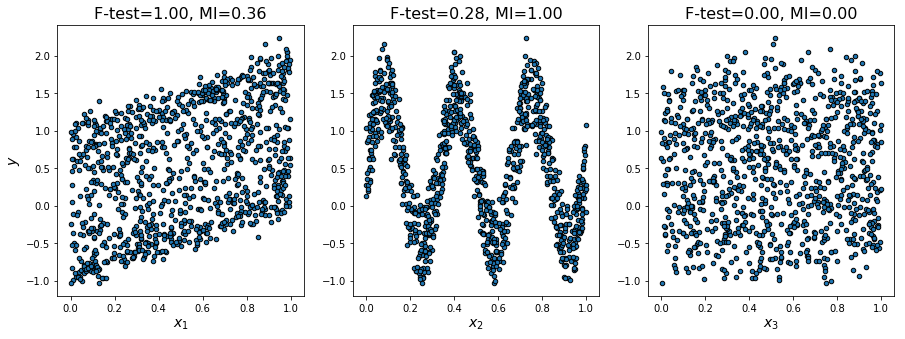其他例子
除了上面的两个例子外,sklearn的官网还给出了一个多项式核的非线性支持向量机的例子Univariate Feature Selection,其证明了单变量特征选择在多项式核的非线性支持向量机的实际作用是让原模型将注意力更多地放在了更重要的特征上,其与多项式核的非线性支持向量机本身所得到的特征重要程度的对比同样有着微妙的联系.原文点这里
参考
sklearn官方文档:Univariate Feature Selectionsklearn官方文档:Comparison of F-test and mutual informationsklearn ApacheCN 官方翻译

转载于:https://www.cnblogs.com/fonttian/p/9162731.html
展开全文• 前言 本文分为四个部分，将按照如下行文顺序进行介绍： Univariate LSTM Models Multivariate LSTM Models Multi-step LSTM Models Multivariate Multi-step LSTM Models 1. 单变量 LSTM 模型（Univariate LSTM ...深度学习 tensorflow keras 时间序列预测 lstm
• proc univariate data=xiti1_1; var x; run; 这个的结果是SAS 系统 UNIVARIATE PROCEDURE 变量: x 矩 N 5 权重总和 5 均值 137 观测总和 685 标准差 7.96868873 方差 63.5 偏度 -0.1828021 峰度 -0.1576043...
• UCR/UEA是时间序列界的“Imagnet”，单变量TSC存档于2018年重新发布，包含128个数据集，Weka格式的ARFF文件（和.txt文件）（约500 MB）第一部分
• 统计分析软件SAS中关于UNIVARIATE过程步的详细解释说明
• Generalized modified least squares in the univariate linear model，刘绪庆，，In this short paper, we mainly aim to appeal to readers to pay close attention to the criterion of modified least squares ...
• 这章我们将这个问题简单地量化为单变量线性回归模型（Univariate linear regression）来理解它。 PS：监督学习最常见的两类问题： 1、回归：预测一个具体的数值输出 2、分类：预测离散值输出 先来看这个...
• FROM :... PROC UNIVARIATE 含七道指令它们的格式如下（每道指令结束后才用逗号分开）： PROC UNIVARIATE 选项串;VAR 变量名称串;BY 变量名称串;FREQ 变量名称;WEIGHT 变量名称;ID 变量名称串;
• 最初是看到这个网站，决心把所有统计分布梳理一遍：Univariate distribution relationships- WM 伯努里分布 | Bernoulli distribution 二项分布 | Binomial distribution Βeta分布 |beta distribution 泊松分布...
• Airbnb 数据集的单变量和双变量分析 这是我在学校的第一个数据科学项目，其中包含所有功能的详细探索性数据分析。 您可以在此存储库中找到 html、rmd 文件。 您可以从 kaggle 源链接中找到数据集： ://...HTML
• 1、一元高斯分布的定义 高斯分布也叫正态分布，主要用于连续变量的分布。假设有一变量，则其高斯分布形式为： 式中是均值（mean），是方差(variance)，方差的平方根叫做标准误（standard deviation），方差的...
• index: proc means | proc freq|proc univariate /*******************proc means*********************/ PROC MEANS <option(s)> <statistic-keyword(s)>; BY <DESCENDING> variable-1 <....
• 1.1 Discrete Random Variables A random variable is discrete if it takes values in some countable set,e.g.,{0,1,2,…}. The mass function(pdf) fX(x)f_X(x)fX​(x) of a discrete random variable XXX is ...
• Feature selection – Part I: univariate selection 特征选择——1：单变量选择 原文链接：http://blog.datadive.net/selecting-good-features-part-i-univariate-selection/ 对于数据科学家或机器学习实践者来...机器学习 特征选择 单变量选择 随机森林 互信息...